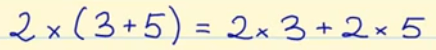Smartick is a fun way to learn math!Aug03

# The Distributive Property of Multiplication

Hello! We’re back again. Today we will look at one of the properties of multiplication: the distributive property of multiplication.

As you may know, multiplication has different properties, let’s review them:

• Commutative property
• Associative property
• Identity Property
• Distributive property

### Let’s focus on the distributive property of multiplication

The distributive property of multiplication states that when a number is multiplied by the sum of two numbers, the first number can be distributed to both of those numbers and multiplied by each of them separately, then adding the two products together for the same result as multiplying the first number by the sum.

Let’s look at the distributive property with this example:According to the distributive property 2 × (3 + 5) will be equal to 2 × 3 + 2 × 5

Let’s check to see if this is true.

2 × (3 + 5) = 2 × 8 = 16

2 × 3 + 2 × 5 = 6 + 10 = 16

In both cases we get the same result, 16, and therefore we can show that the distributive property of multiplication is correct.

I hope that this explanation helps you understand this important property of multiplication.

Go and practice! In Smartick we have lots of exercises for you to learn the best math.

Fun is our brain’s favorite way of learning
Diane Ackerman
Smartick is a fun way to learn math
• 15 fun minutes a day
• Millions of students since 2009• Anguyo GavinMay 29 2021, 2:10 AM

• ApekshaJun 03 2020, 6:37 AM

Nice teaching

• uytrewMay 17 2020, 7:01 PM

GOOD

• KADONGO JAMES KWALIAMar 05 2020, 1:53 PM

As a senior math teacher, the content is well broken down ,organised and explained .I therefore recommend it to be used by students teachers etc.thanks alot to our experts for the continued research.

• SmartickMar 09 2020, 3:32 AM

Thank you very mucho for your kind comment!

• TumiAug 26 2020, 9:43 AM

Nice teaching ,on point

• SksksksksksOct 09 2019, 4:53 PM

How do you do distributive property of multiplication to analyze the equation

• IshanviMay 02 2019, 9:49 PM

I like the way you teach

• jyothinagarajJul 11 2019, 10:23 AM

Nice

• lina jiangSep 28 2019, 12:19 PM

i love math so much that I learned many of them!!!!

• darren jiangSep 28 2019, 12:21 PM

the more learn the better thinking will get